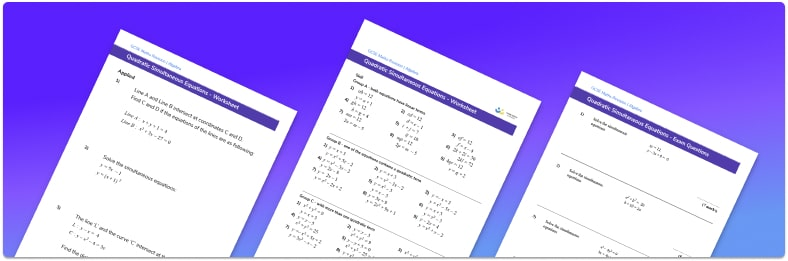• This field is for validation purposes and should be left unchanged.

You can unsubscribe at any time (each email we send will contain an easy way to unsubscribe). To find out more about how we use your data, see our privacy policy.

• Section 1 of the worksheet contains 27 skills-based quadratic simultaneous equations questions, in 3 groups to support differentiation
• Section 2 contains 3 applied quadratic simultaneous equations questions with a mix of worded problems and deeper problem solving questions
• Section 3 contains 4 higher level GCSE exam style questions
• Answers and a mark scheme are provided for all questions
• Questions follow variation theory with plenty of opportunities for students to work independently at their own level
• All practice and exam questions are created by fully qualified expert secondary maths teachers
• Suitable for GCSE maths revision for AQA, OCR and Edexcel exam boards

### Quadratic simultaneous equations at a glance

Quadratic simultaneous equations are pairs of equations where one is one quadratic and one linear.

To solve quadratic simultaneous equations we can use the elimination method in a similar way to solving linear simultaneous equations.  We need to substitute the linear equation into the quadratic (non-linear) equation which may have one variable which is squared, or both variables which are squared. This quadratic equation is then solved and two solutions are found. Both of these solutions need to be substituted one at a time into the first equation or the second equation to calculate two pairs of solutions.

In order to answer these questions, a strong understanding of solving quadratic equations by factorising or using the quadratic formula is required. The final answers can be given as integers, fractions, decimals or surds as required.

Quadratic simultaneous equations can be used to find where a line intersects a parabola or a circle. This can be extended to calculating the equation of a tangent to circle.

Looking forward, students can then progress to additional algebra worksheets, for example a simplifying expressions worksheet, or an inequalities worksheet.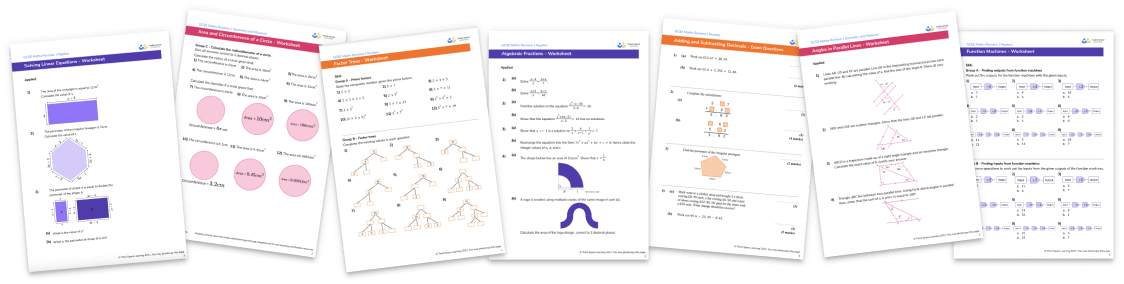For more teaching and learning support on Algebra our GCSE maths lessons provide step by step support for all GCSE maths concepts.

## Related worksheets

Simultaneous Equations Worksheet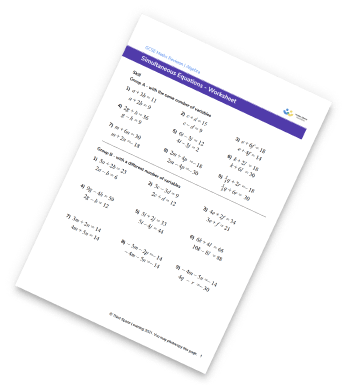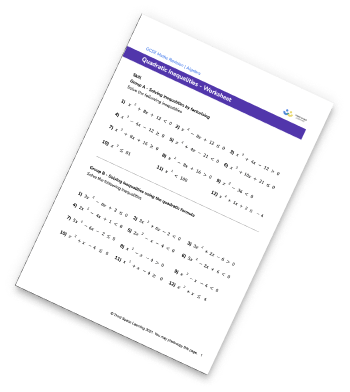Inequalities On A Number Line Worksheet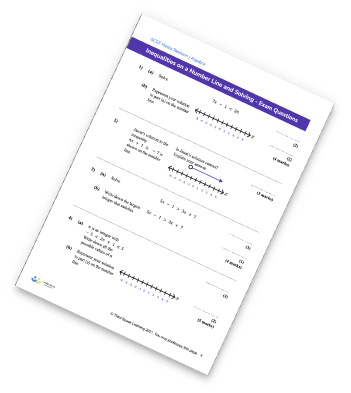Circle Graph Worksheet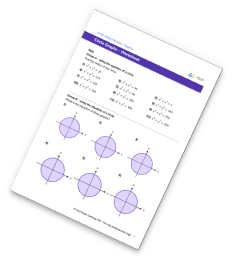## Do you have KS4 students who need more focused attention to succeed at GCSE?There will be students in your class who require individual attention to help them succeed in their maths GCSEs. In a class of 30, it’s not always easy to provide.

Help your students feel confident with exam-style questions and the strategies they’ll need to answer them correctly with our dedicated GCSE maths revision programme.

Lessons are selected to provide support where each student needs it most, and specially-trained GCSE maths tutors adapt the pitch and pace of each lesson. This ensures a personalised revision programme that raises grades and boosts confidence.

Find out more